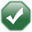# How do I use calculation sequence?

## Summary

This article explains how to use the calculation sequence in a report generator report.

### Where

The Calculation Sequence (Known in the Report Generator as "Calc. Seq.:") is used in the "Column Details" section of Report Creation for column types 'Text', 'Numeric' and 'Date'.

### Default

Each column is given a Calculation Sequence of '50' as default, allowing other columns to be given lower and higher values to help in the order of their evaluation. Note: only the above column types can have their calculation sequence customised.

### Purpose

Each column for the current row will be evaluated in the order dictated by the value entered for the sequence. If columns have the same sequence number, then the column number will be used as the order.A column with a sequence of '00' will be evaluated before a column with a sequence of '01'. If columns 1 and 2 have a sequence of '50', then column 1 will be evaluated before column 2. If column 1 has a sequence of '51' and column 2 has a sequence of '50' then column 1 will be evaluated after column 2.

#### Why go to the Bother?

Seems a lot of bother doesn't it? Why not just keep it set to '50' and forget all about it?

• If you add some columns to an already written report, without the flexibility of this sequence they would always be evaluated after the existing columns. One of these columns may calculate a value that you want to access from an existing column.You add a hidden column 80 that works out the account balance for a date range. You access this from column 5. When column 5 was being added to the report at run time, column 80 wouldn't exist so its value would be '0'... If you altered the sequence of column 80 to a lower value than column 5, when column 5 was being added to the report, column 80 would exist with its value, and that value would be used in column 5.
• Some variables may take a while to evaluate (e.g. Period totals) - these variables hold onto their values until a different period is required, columns that use these should be grouped in sequence.For the "Stock Control Report Generator" variables 'S14', 'S15', 'S16', 'S17', 'S18', 'S19', 'S20', 'S21', 'S22', 'S23', 'S24', 'S25', 'S65' and 'S66' only re-evaluate if the 'Stock Code' or the 'Period' changes. Example 1 You use 'S14{D1}' in column 1. (Sequence of 50). You use 'S14{D2}' in column 2. (Sequence of 50). You use 'S15{D1}' in column 3. (Sequence of 50). The above example would evaluate columns 1, 2 and 3 in that order. It would read all the period history for Date Range 1 twice, once for column 1 and again for column 3 (because the Period Range for each column 2 is different). Example 2 You use 'S14{D1}' in column 1. (Sequence of 50). You use 'S14{D2}' in column 2. (Sequence of 51). You use 'S15{D1}' in column 3. (Sequence of 50). The above example would evaluate columns 1, 2 and 3 in the order of 1, 3 and 2. It would read all the period history for Date Range 1 once (because the Period Range for column 3 is the same as column 1). Result With example 1 the period data is calculated 3 times and for example 2 the period date is calculated 2 times. Depending on the amount of stock history you have this can save massive amounts of time when Platinum is producing the report.

#### Other considerations

If your report is going to screen or printer, the actual information on the report will be laid out according to the "Position:" field of the columns. If you are sending the information to file, the columns will be laid out according to the "Calc. Seq.:" first and then the "Position:" of the column, so your file may not be laid out in the way that you expect.